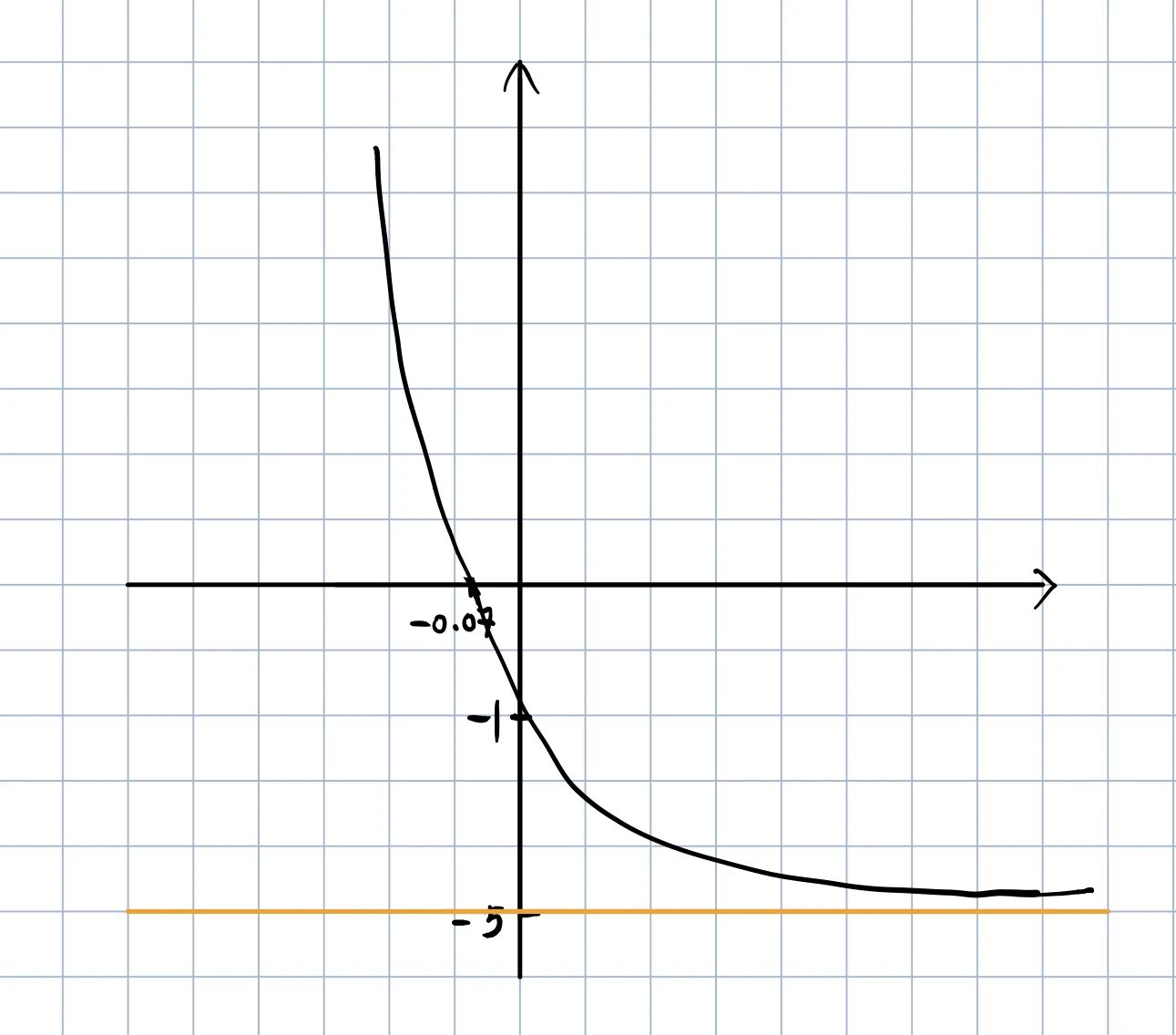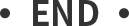# 多维学员专栏 | Additional Maths：Logarithmic and exponential equations# Logarithmic and Exponential Functions

"The book of nature is written in the language of Mathematic"---Galileo

Logarithmic and exponential functions is the 6th chapter of the Additional Mathematics. This post will discuss several identities about logarithms and some exam-style questions.

## Content

1. Definition
2. Graphs
3. Rules of calculation
4. Examination questions

## 1. Definition

Exponential Function (指数函数)：

= (1)

Logarithmic Function (对数函数)：

= (1)

Common Logarithm (常用对数) ：

=

Natural Logarithm (自然对数) ：

=

Euler's number

is one of the most important numbers in mathematics. It can be found in a lot of areas and has a wide range of fascinating properties, which we will discuss later in the article.

is an irrational number and the first few digits are: = 2.718281828459...

We can define in 3 ways:

• Using Limit (极限定义)

=

• Using factorial (阶乘定义)：

= +++++ ...

• Using Euler's Identity (欧拉恒等式) ：

= imginary number

## 2. Graphs

For , the domain is , range is

For , the domain is , range is   ,

• must pass through the point (0,1) because anything to the power of equals to 1

• must pass through the point (1,0) becuase it is an inverse function of

## 3. Laws of Logarithms

There are several laws we have to remember.

Once you understand these laws, it should be pretty easy to do these questions. But please remember, these laws only apply if and are both positive and and 1.

Here are some important laws you must memorize:

• Multiplication:

• Division :

• Power:

• Change of base:

if , then

• Other useful laws:

## 4. Examination Questions

1. Solve the following simultaneous euqations.

Solution:

First, using the rule

,

we can express 2+ into 2.

(Note: can be written as )

Then applying the product rule, this expression can be changed to .

By obeserving both sides, we know .

The second euqation tells us . So now, we have two simplified equations:

It is very simple now when we reach this point. After solving these two simultaneous equations, we can calculate the value of and

From Cambridge IGCSE Additional Mathematics 0606 Paper 21 Q3 Nov 2014

1. Using the sbstitution , solve

Solution:

First, let's express into and can be written as , therefore the left hand side can be rewritten as .

Then, we can substitute into this formula and we will have .

Now, all we need to do is to solve this quardratic equation .

This euqation can be factorised =0, so u =1 or .

Replacing with , we can calculate x or

Cambridge IGCSE Additional Mathematics 0606 Paper 11 Q4 Nov 2012

1. Solve the following equation:

Solution:

First, we will need to change the base of into .

Then, we can simplify the right side to 3+2.(Note: 3 can be rewrite as 3)

Using the multification law, 3+2 can be written as .

Comparing both sides, we can know .

Now it become very easy to solve this quardratic equation, can be factorised to or

1. Sketch the graph of

Solution:

I believe many of the students can do the questions above very easily but they are struggling about drawing graphs.

The very first thing we need to do is to find out the intercepts.

When

y intercept is (0,-1)

When

x intercept is (-0.07,0)

After finding the intercepts, we need to consider the limits.

As so y

Hence the asymptote is -5

As

Using the information above, we can sketch something like this (p.s. this is a very ugly sketch that i've drawn lol):Here is the graph DESMOS create:

https://www.mathsisfun.com/numbers/e-eulers-number.html
Cambridge IGCSE and O level Additional Mathematics Coursebook
Special Thanks：——

A-Level

IGCSE

AP

IB

Sciences

——
AMC
Physics Bowl
BPhO
PUPC
UKChO
CCC
BBO

——

400热线：400 9626 006